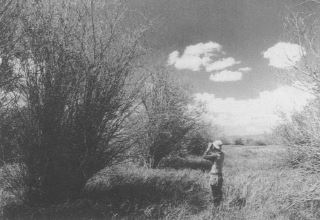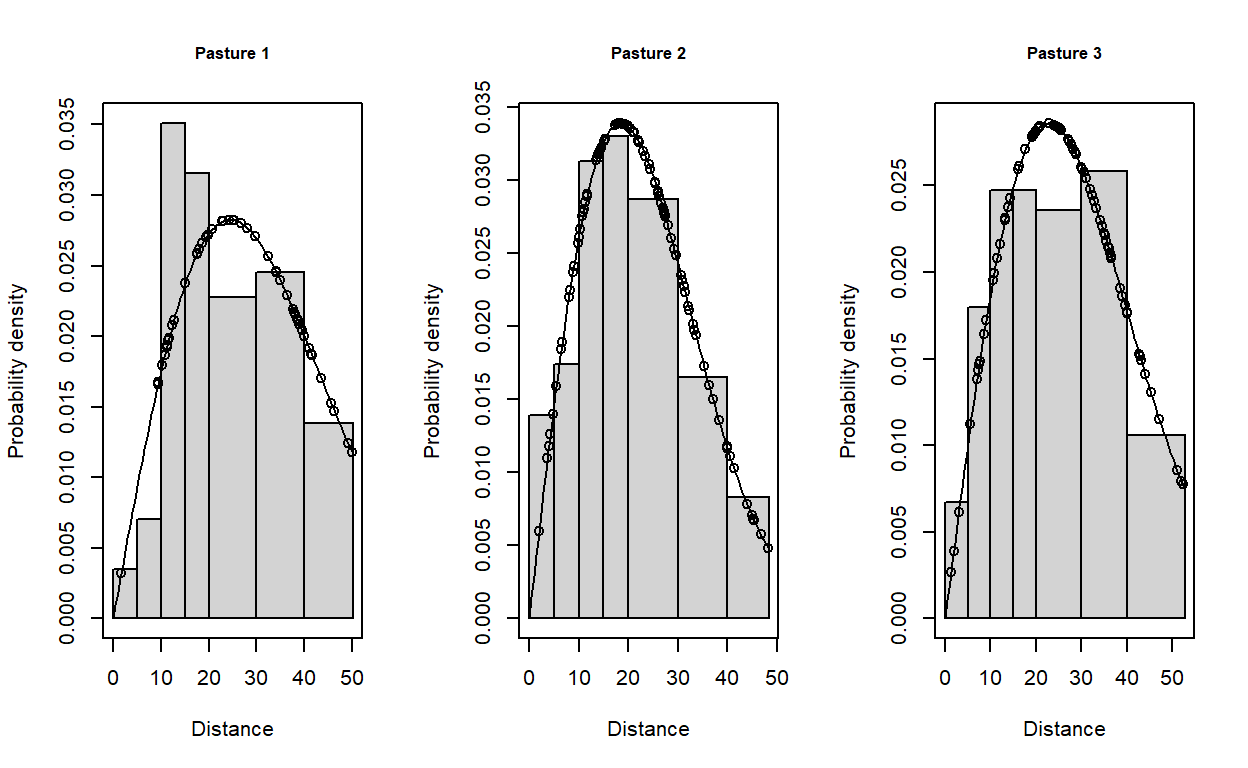# Analysis of stratified survey designs

Revisiting the savanna sparrow point transect data.

Eric Rexstad http://distancesampling.org (CREEM, Univ of St Andrews)https://creem.st-andrews.ac.uk
2022-03-14

In this exercise, we use R and the Distance package to fit different detection function models to point transect survey data of savanna sparrows (Passerculus sandwichensis) density and abundance. These data were part of a study examining the effect of livestock grazing upon vegetation structure and consequently upon the avian community described by Knopf et al. (1988). This dataset was also used to demonstrate point transect analysis

# Objectives

• Fit a detection function pooling data across pastures,
• Fit pasture-specific detection functions,
• Choose most appropriate analysis using model selection.

# Survey design

A total of 373 point transects were placed in three pastures in the Arapaho National Wildlife Refuge in Colorado (Figure 1). Elevation of these pastures was ~2500m. In this example, we will perform pasture-level analysis of these data.Figure 1: Summer grazed pastures along Illinois River Arapaho National Wildlife Refuge, Colorado. Figure from .

The fields of the Savannah_sparrow_1980 data set are:

• Region.Label - three pastures that constituted sections of the study area.
• Area - size of the study region. A place holder, because pasture sizes are not known. Estimates of density and abundance will be equivalent.
• Sample.Label - point transect identifier (total of 273)
• Effort - number of visits to each point
• object - unique identifier for each detected savanna sparrow
• distance - radial distance (metres) to each detection
• Study.Area - only data for savanna sparrow (SASP) are included in this data set

# Make the data available for R session

This command assumes that the dsdata package has been installed on your computer. The R workspace Savannah_sparrow_1980 contains detections of savanna sparrows from point transect surveys of Knopf et al. (1988).

library(Distance)
data(Savannah_sparrow_1980)
conversion.factor <- convert_units("meter", NULL, "hectare")


# Separate data into pasture-specific data sets

The simplest way to fit pasture-specific detection functions is to subset the data. This could be done at the time the ds() function is called, but we perform the step here as a data preparation step.

sasp.past1 <- subset(Savannah_sparrow_1980, Region.Label == "PASTURE 1")
sasp.past2 <- subset(Savannah_sparrow_1980, Region.Label == "PASTURE 2")
sasp.past3 <- subset(Savannah_sparrow_1980, Region.Label == "PASTURE 3")


# Pasture (stratum)-specific detection functions

Fit half-normal key functions without adjustments to each pasture separately after performing 5% right truncation.

past1.hn <- ds(data=sasp.past1, key="hn", adjustment=NULL,
transect="point", convert_units=conversion.factor, truncation="5%")
transect="point", convert_units=conversion.factor, truncation="5%")
transect="point", convert_units=conversion.factor, truncation="5%")


The total AIC for the model that fits separate detection functions to each pasture is the sum of the AICs for the individual pastures.

model.separate.AIC <- sum(AIC(past1.hn, past2.hn, past3.hn)$AIC)  # Common detection function across pastures This model is much simpler to fit because there is only a single call to ds() using the original data. model.pooled <- ds(data=Savannah_sparrow_1980, key="hn", adjustment=NULL, transect="point", convert_units = conversion.factor, truncation = "5%") model.pooled.AIC <- AIC(model.pooled)  # Comparison of AIC scores cat(paste("Stratum-specific detection AIC", round(model.separate.AIC), "\nCommon detection function AIC", round(model.pooled.AIC$AIC)), sep=" ")

Stratum-specific detection AIC 2007
Common detection function AIC 2022

Because the AIC for model with stratum-specific detection functions (2007) is less than AIC for model with pooled detection function (2022), we base our inference upon the stratum-specific detection function model (depicted in Figure 2).

cutpoints <- c(0,5,10,15,20,30,40,53)
par(mfrow=c(1,3))
plot(past1.hn, breaks=cutpoints, pdf=TRUE, main="Pasture 1")
plot(past2.hn, breaks=cutpoints, pdf=TRUE, main="Pasture 2")
plot(past3.hn, breaks=cutpoints, pdf=TRUE, main="Pasture 3")Figure 2: Pasture-specific detection functions based upon half-normal key function.

## Absolute goodness of fit

Always best to check the fit of the preferred model to the data.

gof_ds(past1.hn, plot = FALSE)
gof_ds(past2.hn, plot = FALSE)
gof_ds(past3.hn, plot = FALSE)


Goodness of fit results for ddf object

Distance sampling Cramer-von Mises test (unweighted)
Test statistic = 0.0939637 p-value = 0.615284

Goodness of fit results for ddf object

Distance sampling Cramer-von Mises test (unweighted)
Test statistic = 0.0478569 p-value = 0.889167

Goodness of fit results for ddf object

Distance sampling Cramer-von Mises test (unweighted)
Test statistic = 0.0402974 p-value = 0.931609

Further exploration of analyses involving stratification can be found in the example of dung survey analysis.